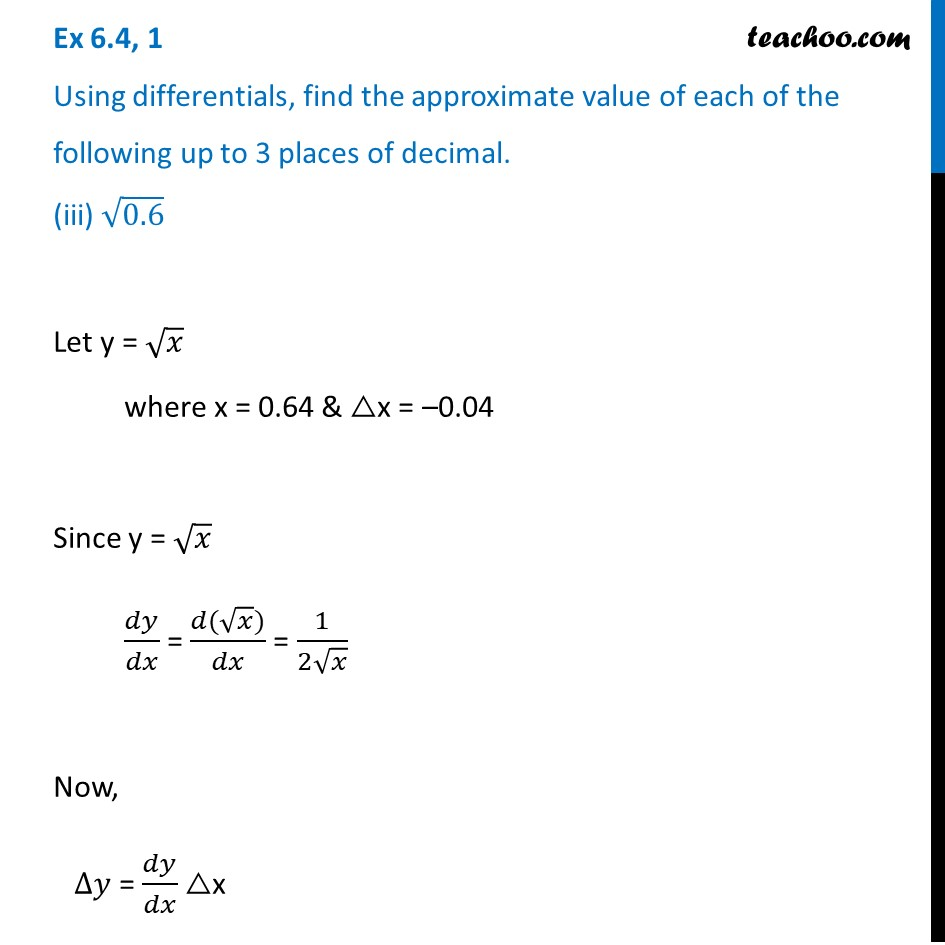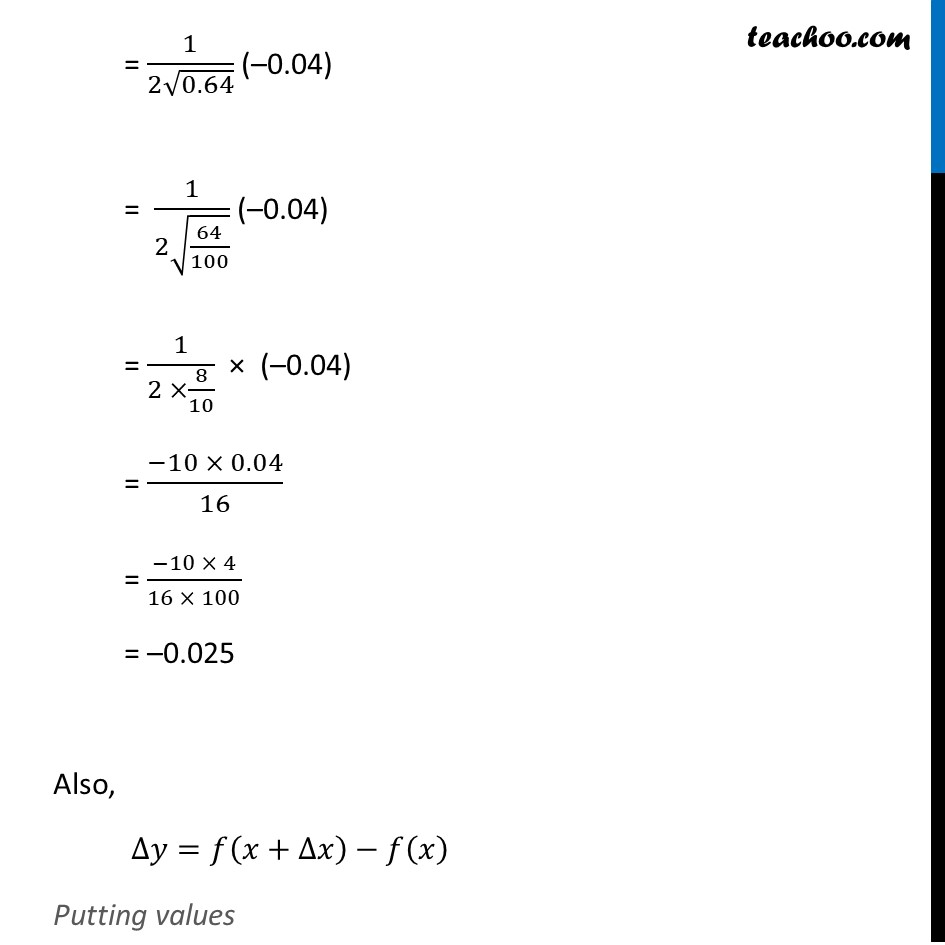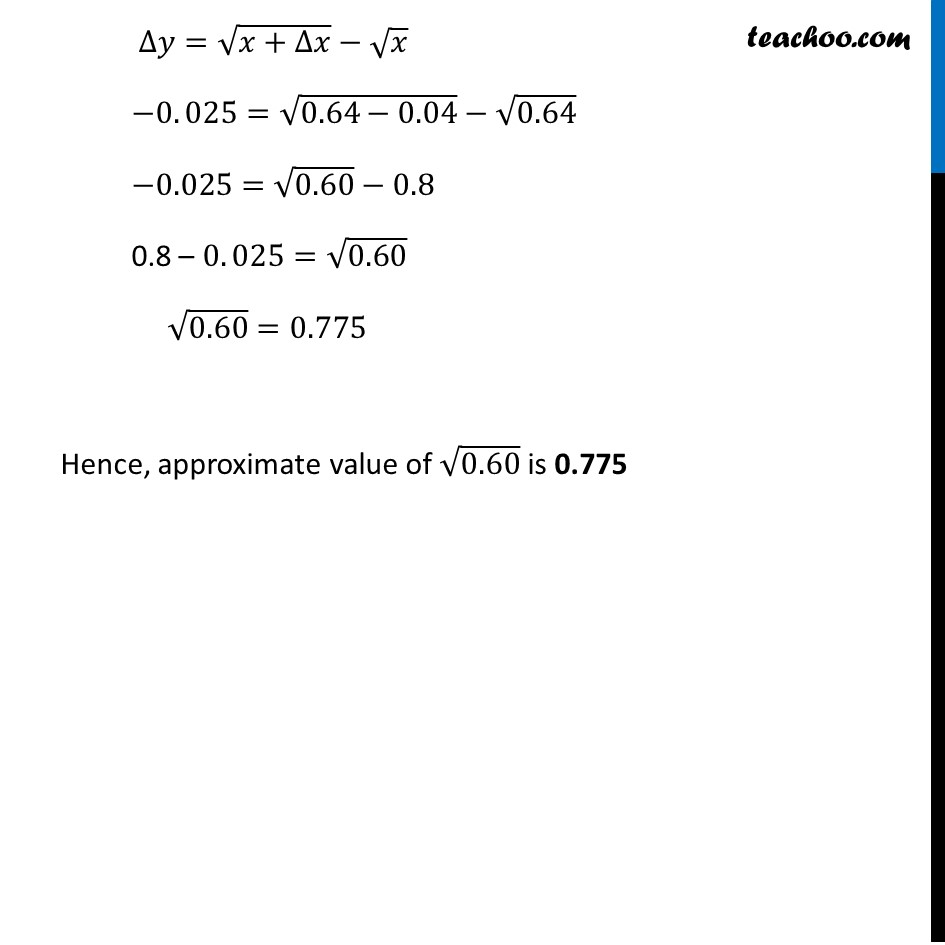Approximations (using Differentiation)

Chapter 6 Class 12 Application of Derivatives
Serial order wiseLearn in your speed, with individual attention - Teachoo Maths 1-on-1 Class

### Transcript

Question 1 Using differentials, find the approximate value of each of the following up to 3 places of decimal. (iii) √0.6 Let y = √𝑥 where x = 0.64 & △x = –0.04 Since y = √𝑥 𝑑𝑦/𝑑𝑥 = (𝑑(√𝑥))/𝑑𝑥 = 1/(2√𝑥) Now, ∆𝑦 = 𝑑𝑦/𝑑𝑥 △x = 1/(2√0.64) (–0.04) = 1/(2√(64/100)) (–0.04) = 1/(2 ×8/10) × (–0.04) = (−10 × 0.04)/16 = (−10 × 4)/(16 × 100) = –0.025 Also, ∆𝑦=𝑓(𝑥+∆𝑥)−𝑓(𝑥) Putting values ∆𝑦=√(𝑥+∆𝑥)−√𝑥 −0. 025=√(0.64−0.04)−√0.64 −0.025=√0.60−0.8 0.8 – 0. 025=√0.60 √0.60=0.775 Hence, approximate value of √0.60 is 0.775